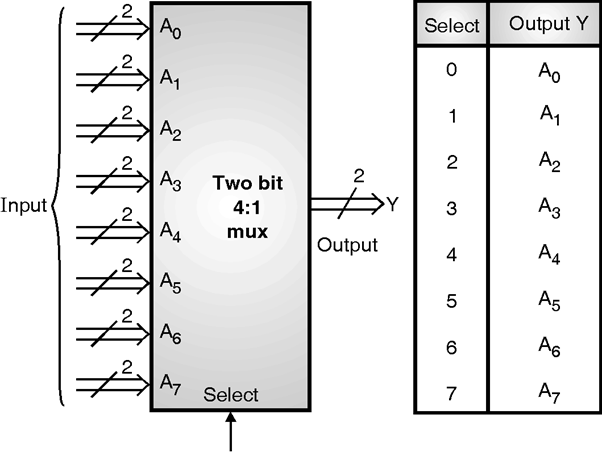Home > VHDL > Logic Circuits > Two bit Wide 8 1 Mux

Implementation of Two-bit Wide 8:1 Mux :1) Using case statement :

library IEEE;

use IEEE.STD_LOGIC_1164.all, IEEE.NUMERIC_STD. all;

entity mux_tbw_case is

port (Sel: in integer range 0 to 7;

A0, A1, A2, A3, A4, A5, A6, A7: in std_logic_vector(1 downto 0);

Y: out std_logic_vector(1 downto 0));

end mux_tbw_case;

architecture behavior of mux_tbw_case is

begin

process (Sel, A0, A1, A2, A3, A4, A5, A6, A7)

begin

case Sel is

when 0 => Y <= A0;

when 1 => Y <= A1;

when 2 => Y <= A2;

when 3 => Y <= A3;

when 4 => Y <= A4;

when 5 => Y <= A5;

when 6 => Y <= A6;

when 7 => Y <= A7;

end case ;

end process;

end behavior ;

2) Using selected signal assignment :

library IEEE;

use IEEE.STD_LOGIC_1164.all, IEEE.NUMERIC_STD. all;

entity mux_tbw_ss is

port (Sel: in integer range 0 to 7;

A0, A1, A2, A3, A4, A5, A6, A7: in std_logic_vector(1 downto 0);

Y: out std_logic_vector(1 downto 0));

end mux_tbw_ss ;

architecture behavior of mux_tbw_ss is

begin

with Sel select

Y <= A0 when 0,

A1 when 1,

A2 when 2,

A3 when 3,

A4 when 4,

A5 when 5,

A6 when 6,

A7 when 7;

end behavior;